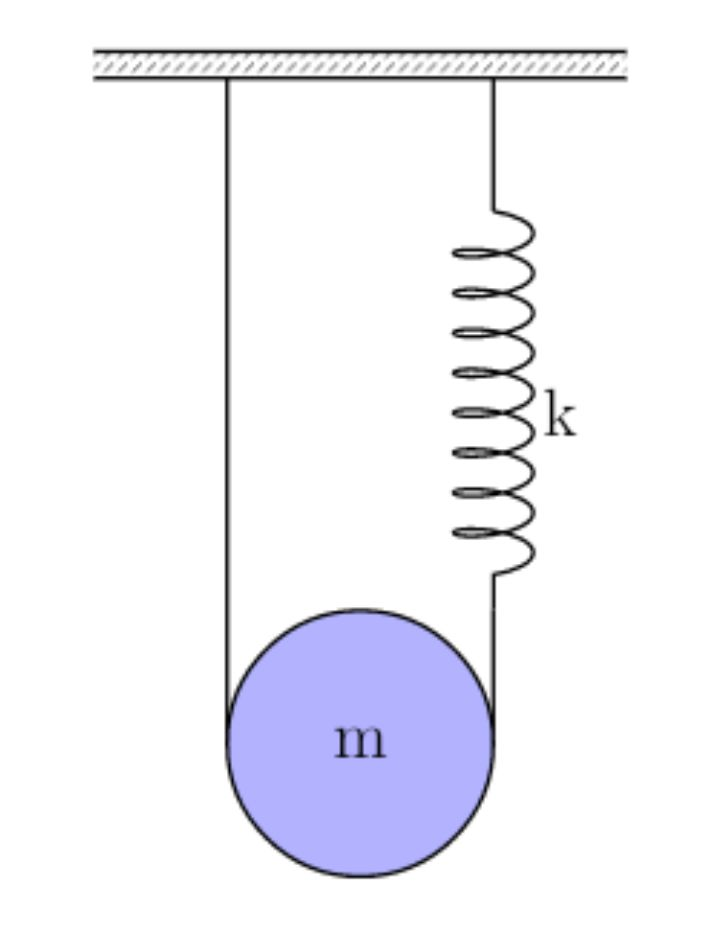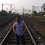# Tessellate S.T.E.M.S - Physics - School - Set 1 - Subjective Problem

A pulley in the form of a circular disc of mass $m$ and radius $r$ has the groove cut all along the perimeter. A string whose one end is attached over to the ceiling passes over this disc pulley and its other end is attached to a spring of spring constant k. The other end of the spring is attached to the ceiling as shown in the figure. Find the time period of vertical oscillations of the centre of mass assuming that the string does not slip over the pulley?This problem is a part of Tessellate S.T.E.M.S.Note by Writabrata Bhattacharya
2 years, 10 months ago

This discussion board is a place to discuss our Daily Challenges and the math and science related to those challenges. Explanations are more than just a solution — they should explain the steps and thinking strategies that you used to obtain the solution. Comments should further the discussion of math and science.

When posting on Brilliant:

• Use the emojis to react to an explanation, whether you're congratulating a job well done , or just really confused .
• Ask specific questions about the challenge or the steps in somebody's explanation. Well-posed questions can add a lot to the discussion, but posting "I don't understand!" doesn't help anyone.
• Try to contribute something new to the discussion, whether it is an extension, generalization or other idea related to the challenge.

MarkdownAppears as
*italics* or _italics_ italics
**bold** or __bold__ bold
- bulleted- list
• bulleted
• list
1. numbered2. list
1. numbered
2. list
Note: you must add a full line of space before and after lists for them to show up correctly
paragraph 1paragraph 2

paragraph 1

paragraph 2

[example link](https://brilliant.org)example link
> This is a quote
This is a quote
    # I indented these lines
# 4 spaces, and now they show
# up as a code block.

print "hello world"
# I indented these lines
# 4 spaces, and now they show
# up as a code block.

print "hello world"
MathAppears as
Remember to wrap math in $$ ... $$ or $ ... $ to ensure proper formatting.
2 \times 3 $2 \times 3$
2^{34} $2^{34}$
a_{i-1} $a_{i-1}$
\frac{2}{3} $\frac{2}{3}$
\sqrt{2} $\sqrt{2}$
\sum_{i=1}^3 $\sum_{i=1}^3$
\sin \theta $\sin \theta$
\boxed{123} $\boxed{123}$

Sort by:

When studying at school, I always do not have enough time for the normal completion of my homework. Sometimes you have to come up with new ways to solve this problem, https://edubirdie.com/words-to-minutes-converter - here you can find words to minutes converter and other useful tools that will help you quickly deal with all problems and save your time.

- 1 year, 1 month ago

2pi_/(3m/8k)

- 2 years, 9 months ago

$\omega ^2=\frac {4kx}{1+\frac {I_{cm}}{mR^2}}$,therefore here $\omega =\sqrt \frac {8k}{3m}$

- 2 years, 9 months ago

how u derived the first formula

- 2 years, 9 months ago

Refer HC Verma. The question had been directly copied from there

- 2 years, 9 months ago

your answer is dimensionally incorrect it would be better if u correct it

- 2 years, 9 months ago

I am not used to doing such mistakes.Kindly check it correctly the answer is correct as are the dimensions.

- 2 years, 9 months ago

π(m/k)^(1/2)

- 2 years, 10 months ago